Updating search results...

# 23 Results

View
Selected filters:
• MCCRS.Math.Content.7.G.B.5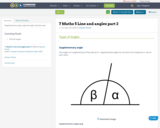Conditional Remix & Share Permitted
CC BY-SA
Rating
0.0 stars

Supplementary angle, adjacent angle and linear pair

Subject:
Mathematics
Material Type:
01/28/2016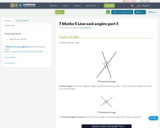Conditional Remix & Share Permitted
CC BY-SA
Rating
0.0 stars

Parallel Line

Subject:
Mathematics
Material Type:
01/28/2016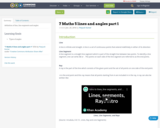Conditional Remix & Share Permitted
CC BY-SA
Rating
0.0 stars

definition of line, line segments and angles

Subject:
Mathematics
Material Type:
01/28/2016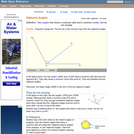Rating
0.0 stars

An interactive applet and associated web page that show the concept of adjacent angles (two angles that share a common leg). The applet shows three line segments with a common endpoint. The user can move the center one and see that the angles on both sides (the adjacent angles) of it are affected. Applet can be enlarged to full screen size for use with a classroom projector. After use in the classroom, students can access it again from any web browser at home or in the library with no login required. This resource is a component of the Math Open Reference Interactive Geometry textbook project at http://www.mathopenref.com.

Subject:
Geometry
Mathematics
Material Type:
Simulation
Provider:
Math Open Reference
Author:
John Page
02/16/2011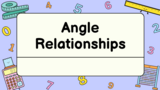Unrestricted Use
CC BY
Rating
0.0 stars

This OER explores the angle relationships using inquiry-based methods. The included slideshow has multiple examples and practice problems, as well as notes for student to take.&nbsp;

Subject:
Mathematics
Material Type:
Lecture Notes
Author:
Sara Parker
06/28/2023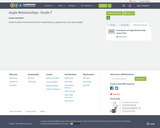Conditional Remix & Share Permitted
CC BY-NC
Rating
0.0 stars

Students explore the characteristics of complementary, supplementary, and vertical angles.

Subject:
Mathematics
Material Type:
Lesson Plan
Author:
Becky Caya
mike simons
01/27/2018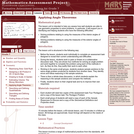Only Sharing Permitted
CC BY-NC-ND
Rating
0.0 stars

This lesson unit is intended to help you assess how well students are able to use geometric properties to solve problems. In particular, it will support you in identifying and helping students who have the following difficulties: Solving problems relating to using the measures of the interior angles of polygons; and solving problems relating to using the measures of the exterior angles of polygons.

Subject:
Geometry
Mathematics
Material Type:
Assessment
Lesson Plan
Provider:
Shell Center for Mathematical Education
Provider Set:
Mathematics Assessment Project (MAP)
04/26/2013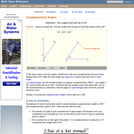Rating
0.0 stars

An interactive applet and associated web page that demonstrate the concept of complementary angles (angles that add to 90 degrees). The applet shows two angles. You can drag the endpoints of each angle and the other angle changes so that they always add to 90 degrees. They are drawn in such a way that it is visually obvious that together they form a right angle, although they are separate on the page. The angle measure readouts can be turned off for class discussions. Applet can be enlarged to full screen size for use with a classroom projector. This resource is a component of the Math Open Reference Interactive Geometry textbook project at http://www.mathopenref.com.

Subject:
Geometry
Mathematics
Material Type:
Simulation
Provider:
Math Open Reference
Author:
John Page
02/16/2011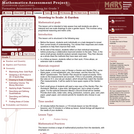Only Sharing Permitted
CC BY-NC-ND
Rating
0.0 stars

This lesson unit is intended to help assess how well students are able to interpret and use scale drawings to plan a garden layout. This involves using proportional reasoning and metric units.

Subject:
Algebra
Geometry
Mathematics
Ratios and Proportions
Material Type:
Assessment
Lesson Plan
Provider:
Shell Center for Mathematical Education
Provider Set:
Mathematics Assessment Project (MAP)
04/26/2013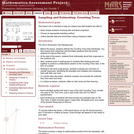Only Sharing Permitted
CC BY-NC-ND
Rating
0.0 stars

This lesson unit is intended to help you assess how well students are able to: solve simple problems involving ratio and direct proportion; choose an appropriate sampling method; and collect discrete data and record them using a frequency table.

Subject:
Education
Geometry
Mathematics
Measurement and Data
Ratios and Proportions
Material Type:
Assessment
Lecture Notes
Lesson Plan
Teaching/Learning Strategy
Provider:
Shell Center for Mathematical Education
Provider Set:
Mathematics Assessment Project (MAP)
Author:
http://map.mathshell.org/
04/26/2013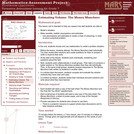Only Sharing Permitted
CC BY-NC-ND
Rating
0.0 stars

This lesson unit is intended to help you assess how well students are able to: Model a situation; make sensible, realistic assumptions and estimates; and use assumptions and estimates to create a chain of reasoning, in order to solve a practical problem.

Subject:
Geometry
Mathematics
Material Type:
Assessment
Lesson Plan
Provider:
Shell Center for Mathematical Education
Provider Set:
Mathematics Assessment Project (MAP)
04/26/2013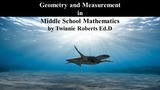Unrestricted Use
CC BY
Rating
0.0 stars

This supplemental resource provides problems and activities related to&nbsp;Geometry and Measurement in Middle School Mathematics.&nbsp;

Subject:
Mathematics
Material Type:
Activity/Lab
Author:
Twianie Roberts Ed.D
09/25/2022Educational Use
Rating
0.0 stars

Students learn about radar imaging and its various military and civilian applications that include recognition and detection of human-made targets, and the monitoring of space, deforestation and oil spills. They learn how the concepts of similarity and scaling are used in radar imaging to create three-dimensional models of various targets. Students apply the critical attributes of similar figures to create scale models of a radar imaging scenario using infrared range sensors (to emulate radar functions) and toy airplanes (to emulate targets). They use technology tools to measure angles and distances, and relate the concept of similar figures to real-world applications.

Subject:
Education
Material Type:
Activity/Lab
Provider:
TeachEngineering
Provider Set:
TeachEngineering
Author:
Mounir Ben Ghalia
Rocio Denise Nava
10/14/2015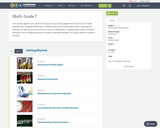Conditional Remix & Share Permitted
CC BY-NC
Rating
0.0 stars

Four full-year digital course, built from the ground up and fully-aligned to the Common Core State Standards, for 7th grade Mathematics. Created using research-based approaches to teaching and learning, the Open Access Common Core Course for Mathematics&nbsp;is designed with student-centered learning in mind, including activities for students to develop valuable 21st century skills and academic mindset.

Subject:
Mathematics
Material Type:
Full Course
Provider:
Pearson
10/06/2016Conditional Remix & Share Permitted
CC BY-NC
Rating
0.0 stars

Constructions and Angles

Unit Overview

Type of Unit: Concept

Prior Knowledge

Students should be able to:

Use a protractor and ruler.
Identify different types of triangles and quadrilaterals and their characteristics.

Lesson Flow

After an initial exploratory lesson involving a paper folding activity that gets students thinking in general about angles and figures in a context, the unit is divided into two concept development sections. The first section focuses on types of angles—adjacent, supplementary, complementary, and vertical—and how they are manifested in quadrilaterals. The second section looks at triangles and their properties, including the angle sum, and how this affects other figures.

In the first set of conceptual lessons, students explore different types of angles and where the types of angles appear in quadrilaterals. Students fold paper and observe the angles formed, draw given angles, and explore interactive sketches that test many cases. Students use a protractor and ruler to draw parallelograms with given properties. They explore sketches of parallelograms with specific properties, such as perpendicular diagonals. After concluding the investigation of the angle types, students move on to the next set.

In the second set of conceptual development lessons, students focus on triangles. Students again fold paper to create figures and certain angles, such as complementary angles.

Students draw, using a protractor and ruler, other triangles with given properties. Students then explore triangles with certain known and unknown elements, such as the number of given sides and angles. This process starts with paper folding and drawing and continues with exploration of interactive sketches. Students draw conclusions about which cases allow 0, 1, 2, or an infinite number of triangles. In the course of the exploration, students discover that the sum of the measure of the interior angles of a triangle is 180°. They also learn that the sum of the measures of the interior angles of a quadrilateral is 360°. They explore other polygons to find their angle sum and determine if there is a relationship to angle sum of triangles. The exploration concludes with finding the measure of the interior angles of regular polygons and speculating about how this relates to a circle.

Lastly, students solve equations to find unknown angle measures. Using their previous experience, students find the remaining angle measures in a parallelogram when only one angle measure is given. Students also play a game similar to 20 Questions to identify types of triangles and quadrilaterals. Having completed the remaining lessons, students have a four-day Gallery to explore a variety of problems.

The unit ends with a unit assessment.

Subject:
Geometry
Mathematics
Material Type:
Unit of Study
Provider:
Pearson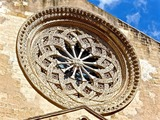Conditional Remix & Share Permitted
CC BY-NC
Rating
0.0 stars

Lesson OverviewStudents explore different polygons by drawing them, measuring angles, and manipulating interactive sketches to find the angle sum for any polygon. Students also explore the angle measures in regular polygons.Key ConceptsThe angle sum in a triangle is 180&deg;. A quadrilateral can be composed of two triangles, so the angle sum of a quadrilateral is 360&deg;.The number of triangles that compose a polygon is two less than the number of sides (angles). The sum of the interior angles in a polygon with n sides is 180&deg; (n &ndash; 2).Goals and Learning ObjectivesFind angle sums in polygons.Generalize to find the angle sum for any polygon.Find interior angle measures for regular polygons.&nbsp;

Subject:
Geometry
Material Type:
Lesson Plan
09/21/2015Conditional Remix & Share Permitted
CC BY-NC
Rating
0.0 stars

Students learn about four types of angles: adjacent, vertical, supplementary, and complementary. They explore the relationships between these types of angles by folding paper, measuring angles with a protractor, and exploring interactive sketches.Key ConceptsAdjacent angles are two angles that share a common vertex and a common side, but do not overlap. Angles 1 and 2 are adjacent angles.Supplementary angles are two angles whose measures have a sum of 180&deg;. Angles 3 and 4 are supplementary angles. Complementary angles are two angles whose measures have a sum of 90&deg;. Angles 5 and 6 are complementary angles. Vertical angles are the opposite angles formed by the intersection of two lines. Vertical angles are congruent. Angles 1 and 2 are vertical angles. Angles 3 and 4 are also vertical angles.Goals and Learning ObjectivesMeasure angles with a protractor and estimate angle measures as greater than or less than 90&deg;.Understand the definition of vertical, adjacent, supplementary, and complementary angles.Explore the relationships between these types of angles.

Subject:
Geometry
Material Type:
Lesson Plan
09/21/2015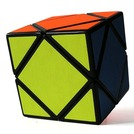Conditional Remix & Share Permitted
CC BY-NC
Rating
0.0 stars

Students solve for missing angle measures by applying what they have learned about types of angles and the angle measures of polygons. Students do a pre-assessment at the end of the lesson.Key ConceptsThere are many defining characteristics for angles, triangles, quadrilaterals, and polygons. Students have discovered these properties throughout this unit and have investigated why they are true. These characteristics and properties will be looked at more formally in high school geometry.Goals and Learning ObjectivesSolve for missing angle measures in polygons.

Subject:
Geometry
Material Type:
Lesson Plan
09/21/2015Conditional Remix & Share Permitted
CC BY-NC
Rating
0.0 stars

Students critique and revise their work from the Self Check after receiving feedback. Students then take a quiz to review the goals of the unit.Key ConceptsStudents reflect on their work and apply what they&#39;ve learned about the characteristics of geometric figures.Goals and Learning ObjectivesCritique and revise work on the Self Check.Apply skills learned in the unit.Understand the relationship of angles:Created by intersecting lines.Found in quadrilaterals, triangles, and polygons.

Subject:
Geometry
Material Type:
Lesson Plan
09/21/2015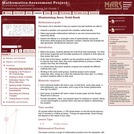Only Sharing Permitted
CC BY-NC-ND
Rating
0.0 stars

This lesson unit is intended to help you assess how well students are able to: Interpret a situation and represent the variables mathematically; select appropriate mathematical methods to use; explore the effects on the area of a rectangle of systematically varying the dimensions whilst keeping the perimeter constant; interpret and evaluate the data generated and identify the optimum case; and communicate their reasoning clearly.

Subject:
Geometry
Mathematics
Material Type:
Assessment
Lesson Plan
Provider:
Shell Center for Mathematical Education
Provider Set:
Mathematics Assessment Project (MAP)